Area - Important Formulae of the Area - Area Bounded By Curve

Area - Area Bounded By Curve

1.

The area bounded by the continuous curve y = f(x), the axis of x and the ordinates x = a and x = b (where b>a and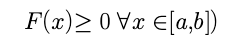is given by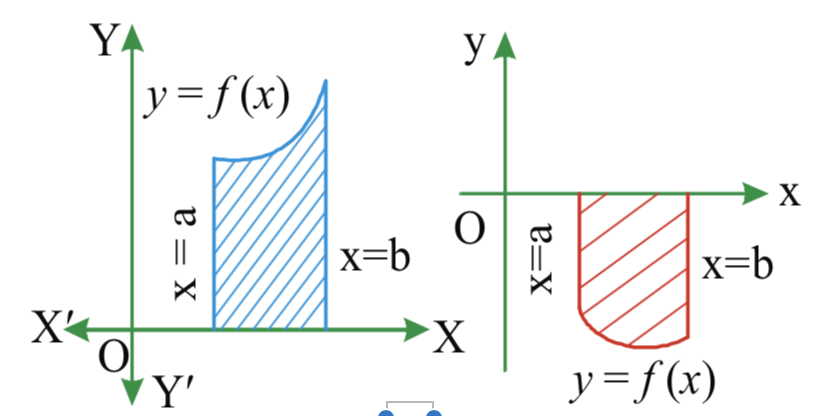2.

If the curve cross the x- axis at (c, 0) then the area bounded by the curve y = f(x)and the coordinate x = a x = b(b > a and a < c < b) is given by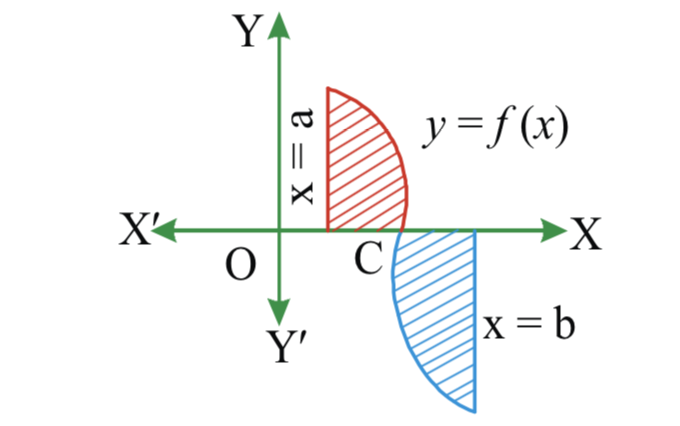3.

When two curves y =f (x) and y =g (x) intersect, the bounded areawhere and b are roots of equation f(x)  g(x) where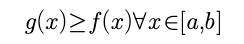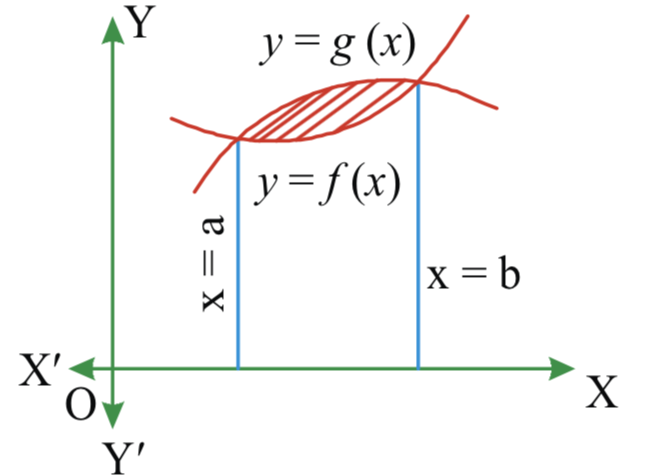4.

The area bound by the straight lines x=a, x=b(a < b) and the curves y=f(x) andy=g(x) Provided f(x)<g(x (a<= x<=b), is given by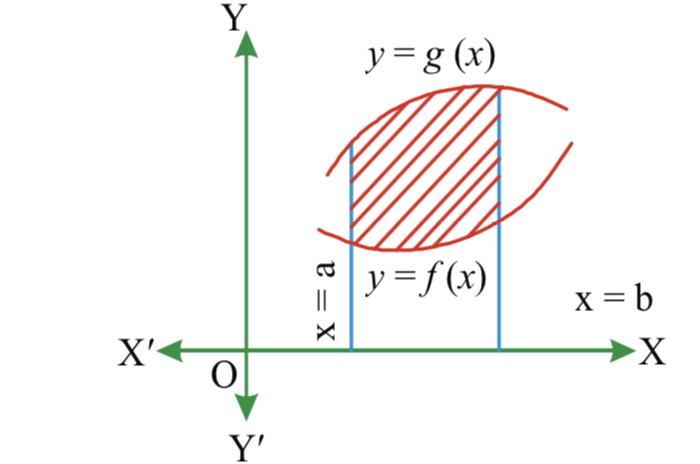5.

Formulae :

1.

The area of rhombus formed by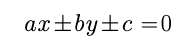is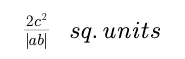2. The area of the region bounded by |x| + |y | =.  a is. 2a*a sq.units

3.   The area of the triangle formed by the lines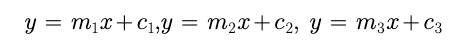is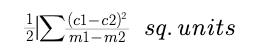4.  The area of the parallelogram formed by a1x+ b1y +c1 = 0, a1x + b1y +d1  = 0, a2x. + b2y +  c2 = 0,a2x + b2y + d2 = 0. is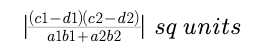5. Area of ther egion bounded by y = ax2 + bx + c. and x -axis  is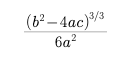Post By : Rahul Kumar 09 Apr, 2020 1570 views Maths